Ex 10.3

Chapter 10 Class 11 Straight Lines
Serial order wise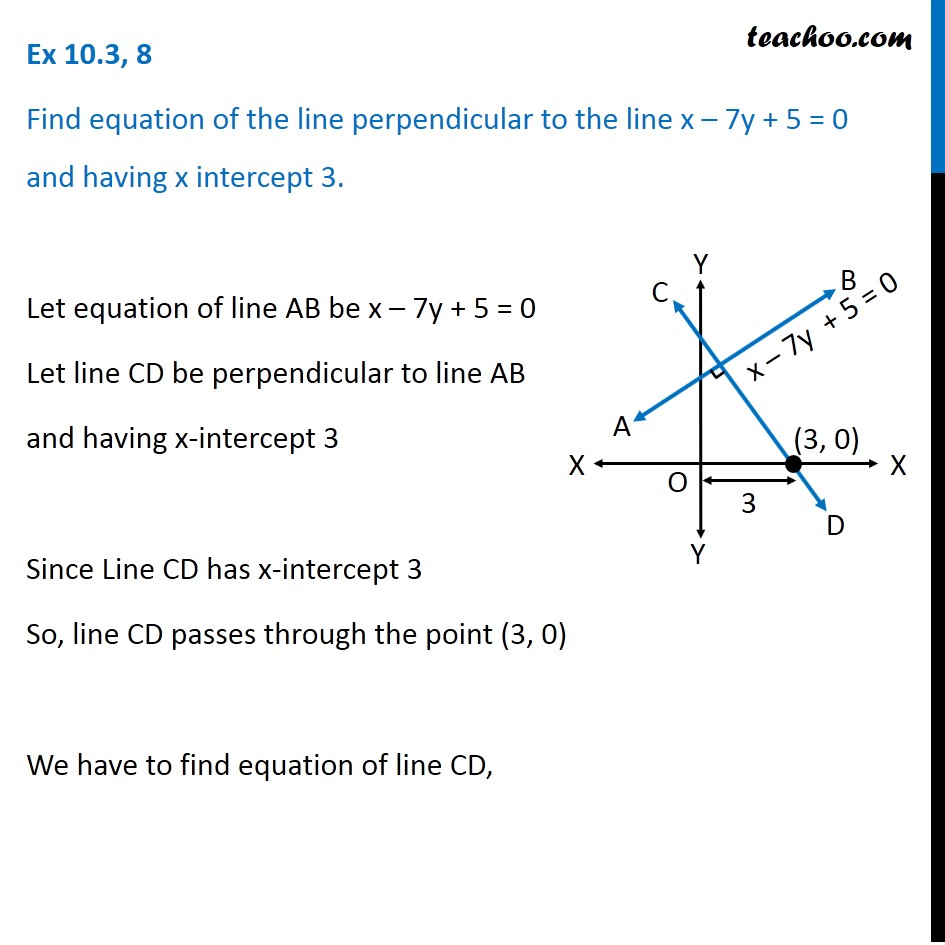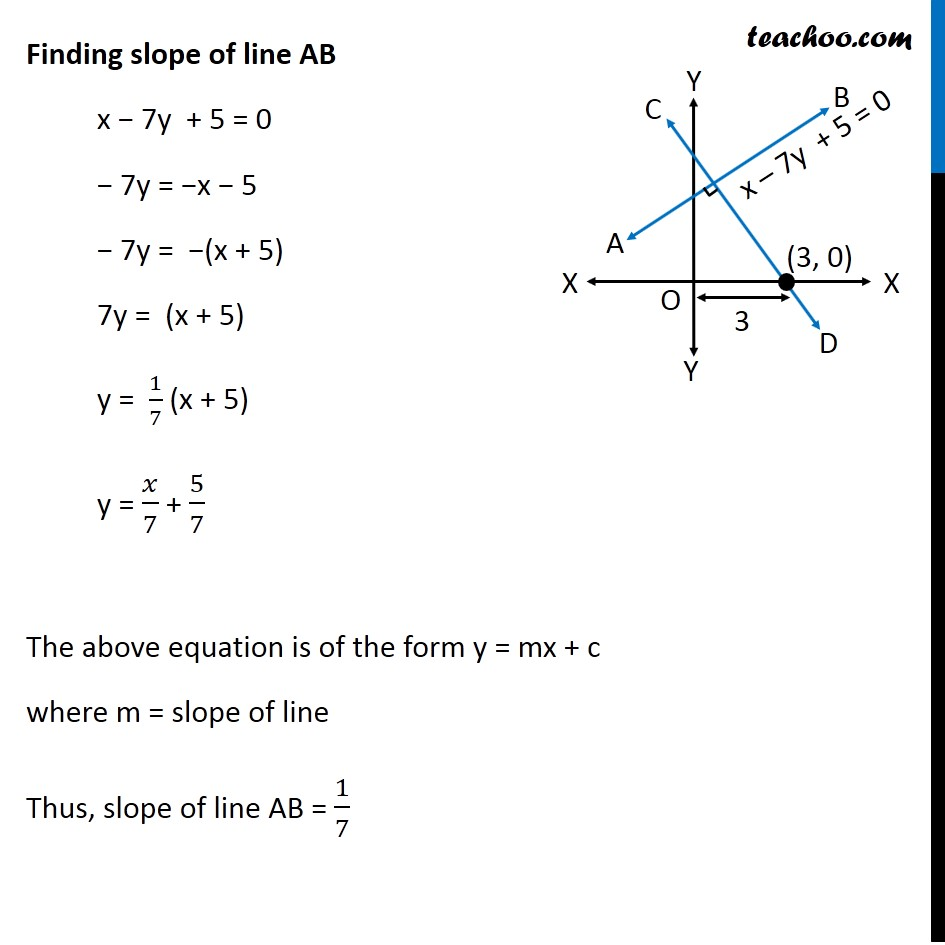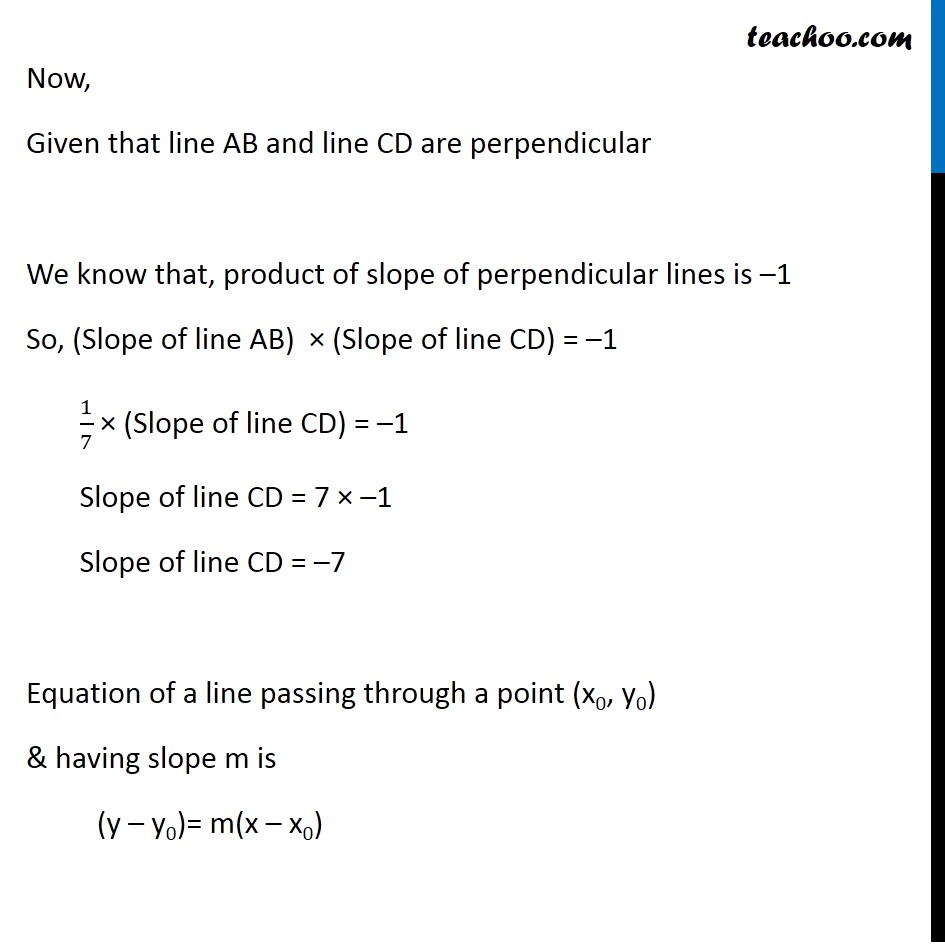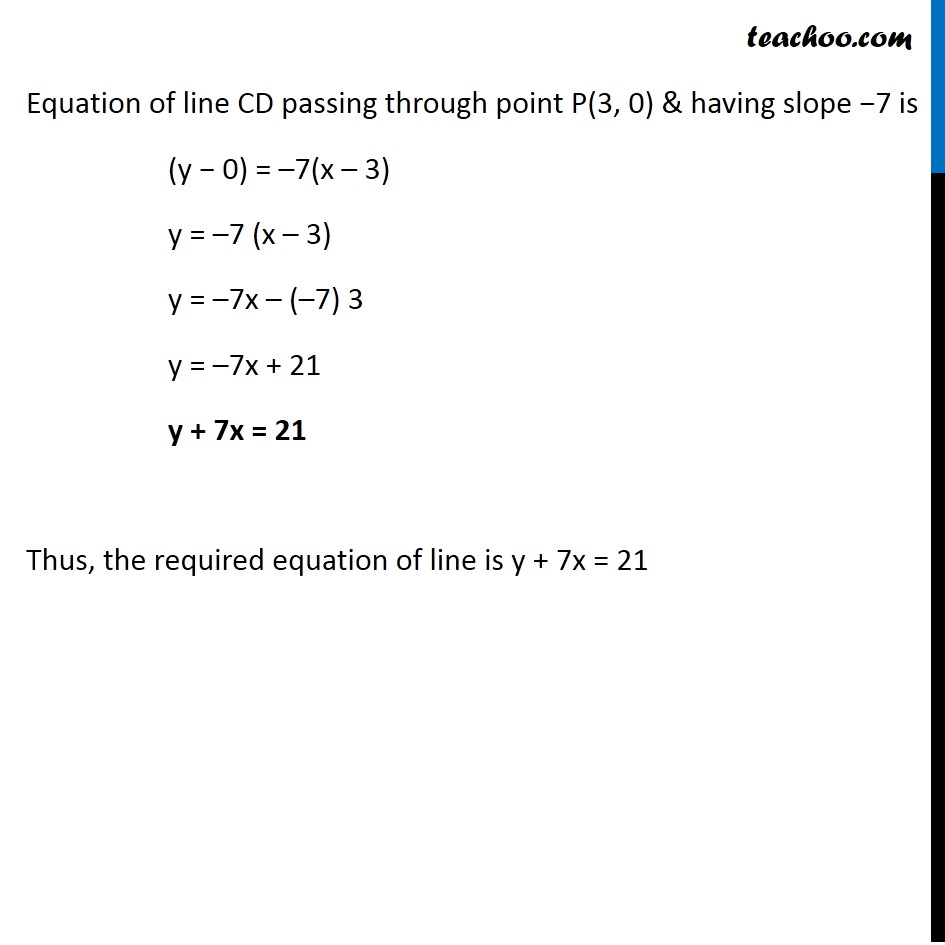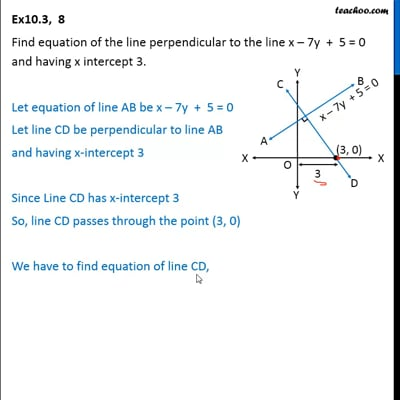This video is only available for Teachoo black users

Solve all your doubts with Teachoo Black (new monthly pack available now!)

### Transcript

Ex 10.3, 8 Find equation of the line perpendicular to the line x – 7y + 5 = 0 and having x intercept 3. Let equation of line AB be x – 7y + 5 = 0 Let line CD be perpendicular to line AB and having x-intercept 3 Since Line CD has x-intercept 3 So, line CD passes through the point (3, 0) We have to find equation of line CD, Finding slope of line AB x − 7y + 5 = 0 − 7y = −x − 5 − 7y = −(x + 5) 7y = (x + 5) y = 1/7 (x + 5) y = 𝑥/7 + 5/7 The above equation is of the form y = mx + c where m = slope of line Thus, slope of line AB = 1/7 Now, Given that line AB and line CD are perpendicular We know that, product of slope of perpendicular lines is –1 So, (Slope of line AB) × (Slope of line CD) = –1 1/7 × (Slope of line CD) = –1 Slope of line CD = 7 × –1 Slope of line CD = –7 Equation of a line passing through a point (x0, y0) & having slope m is (y – y0)= m(x – x0) Equation of line CD passing through point P(3, 0) & having slope −7 is (y − 0) = –7(x – 3) y = –7 (x – 3) y = –7x – (–7) 3 y = –7x + 21 y + 7x = 21 Thus, the required equation of line is y + 7x = 21